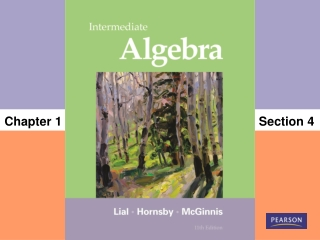DownloadDownload PresentationSection 4

# Section 4

Download Presentation## Section 4

- - - - - - - - - - - - - - - - - - - - - - - - - - - E N D - - - - - - - - - - - - - - - - - - - - - - - - - - -
##### Presentation Transcript

1. Chapter 1 Section 4

2. Properties of Real Numbers Use the distributive property. Use the identity properties. Use the inverse properties. Use the commutative and associative properties. Use the multiplication property of 0. 1.4

3. Use the distributive property. Objective 1 Slide 1.4- 3

4. Use the distributive property. The distributive property can be extended to more than two numbers and provides a way to rewrite a product as a sum. a(b + c + d) = ab + ac + ad When we rewrite a(b + c) as ab + ac, we sometimes refer to the process as “removing” or “clearing” parentheses. Slide 1.4- 4

5. Use the distributive property to rewrite each expression. 4(p – 5) =  4p + 20 6m + 2m =  6m + 2m =  4m CLASSROOM EXAMPLE 1 Using the Distributive Property Solution: =  4p  (4)(5) = (6 + 2)m Slide 1.4- 5

6. 2r + 3s Because there is no common number or variable here, we cannot use the distributive property to rewrite the expression. 5(4p – 2q + r) = = 20p – 10q + 5r CLASSROOM EXAMPLE 1 Using the Distributive Property (cont’d) Solution: 5(4p)  5(2q) + 5r Slide 1.4- 6

7. Use the identity properties. Objective 2 Slide 1.4- 7

8. Use the identity properties. The number 0 is the only number that can be added to any number and leaves the number unchanged. Thus, zero is called the identity elementfor addition, or the additive identity. Similarly, the number 1 is the only number that can be multiplied with another number and leaves the number unchanged. Thus, one is called the identity elementfor multiplication or the multiplicative identity. Slide 1.4- 8

9. Simplify each expression. x – 3x = 1x – 3x = 1x – 3x Identity property. = (1 – 3)x Distributive property. = 2x Subtract inside parentheses. (3 + 4p) = 1(3 + 4p) = 1(3) + (1)(4p)Identity property. = 3 – 4p Multiply. CLASSROOM EXAMPLE 2 Using the Identity Property 1 ∙ a = a Solution: Slide 1.4- 9

10. Use the inverse properties. Objective 3 Slide 1.4- 10

11. The multiplicative inverse (or reciprocal) of a number a is Multiplicative inverses have a product of 1. Use the inverse properties. The additive inverse (or opposite) of a number a is − a. Additive inverses have a sum of 0. The inverse properties “undo” addition or multiplication. Slide 1.4- 11

12. A termis a number or the product of a number and one or more variables raised to powers. The numerical factor in a term is called the numerical coefficient, or just the coefficient. Terms with exactly the same variables raised to exactly the same powers are called like terms. 5y and 21y6x2 and 9x2 Like terms 3m and 16x 7y3 and 3y2 Unlike terms Remember that only like terms may be combined. Use the inverse properties. Slide 1.4- 12

13. Use the commutative and associative properties. Objective 4 Slide 1.4- 13

14. Use the commutative and associative properties. Commutative properties Associative properties Slide 1.4- 14

15. Simplify. 12b – 9 + 4b – 7b + 1 = (12b + 4b) – 9 – 7b + 1 = (12 + 4)b – 9 – 7b + 1 = 16b – 9 – 7b + 1 = (16b – 7b) – 9 + 1 = (16 – 7)b – 9 + 1 = 9b – 8 CLASSROOM EXAMPLE 3 Using the Commutative and Associative Properties Solution: Slide 1.4- 15

16. Simplify each expression. 12b – 9b + 5b – 7b = (12 – 9 + 5 – 7)bDistributive property. = bCombine like terms. 6 – (2x + 7) – 3 = 6 – 2x – 7 – 3 Distributive property. = –2x + 6 – 7 – 3 Commutative property. = –2x – 4 Combine like terms. CLASSROOM EXAMPLE 4 Using the Properties of Real Numbers Solution: Slide 1.4- 16

17. 4m(2n) = (4)(2)mn = 8mn CLASSROOM EXAMPLE 4 Using the Properties of Real Numbers (cont’d) Solution: Slide 1.4- 17

18. Use the multiplication property of 0. Objective 5 Slide 1.4- 18

19. Use the multiplication property of 0. Slide 1.4- 19